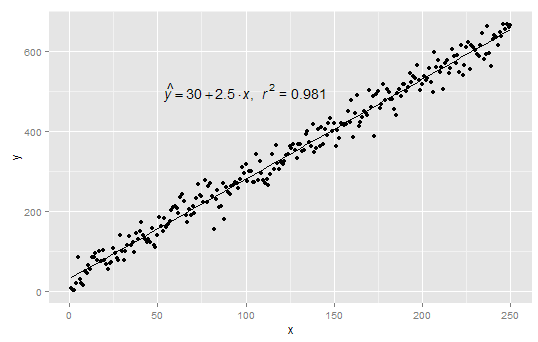# R : Add Linear Regression Equation and RSquare to Graph

In this article, we would see how to add linear regression equation and r-squared to a graph in R. It is very useful when we need to document or present our statistical results. Many people are familiar with R-square as a performance metrics for linear regression. If you are novice in linear regression technique, you can read this article - Linear Regression with R

Create Sample Data

The following program prepares data that is used to demonstrate the method of adding regression equation and rsquare to graph.
x = c(1:250)
mydata= data.frame(x, y= 30 + 2.5 * x + rnorm(250,sd = 25))
library(ggplot2)
R Function
linear = function(k) {
z <- list(xx = format(coef(k), digits = 2),
yy = format(abs(coef(k)), digits = 2),
r2 = format(summary(k)\$r.squared, digits = 3));
if (coef(k) >= 0)  {
eq <- substitute(italic(hat(y)) == xx + yy %.% italic(x)*","~~italic(r)^2~"="~r2,z)
} else {
eq <- substitute(italic(hat(y)) == xx - yy %.% italic(x)*","~~italic(r)^2~"="~r2,z)
}
as.character(as.expression(eq));
}
fo = y ~ x
linplot <- ggplot(data = mydata, aes(x = x, y = y)) + geom_smooth(method = "lm", se=FALSE, color="black", formula = fo) +  geom_point()
linplot1 = linplot + annotate("text", x = 100, y = 500, label = linear(lm(fo, mydata)), colour="black", size = 5, parse=TRUE)
linplot1Regression Line
Related Posts